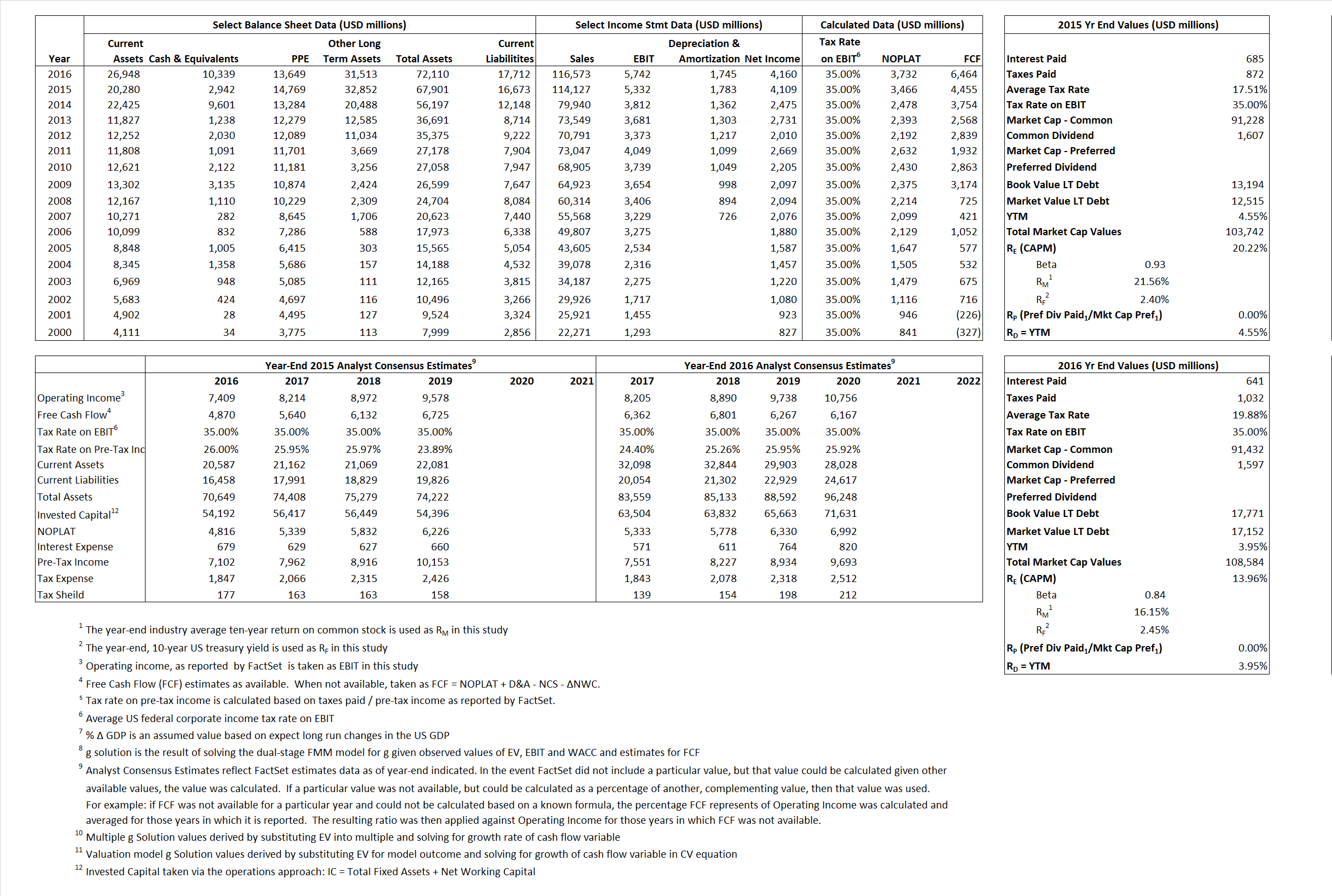# Walgreens Boots Alliance

## Analyst Listing

The following analysts provide coverage for the subject firm as of May 2016:

 Broker Analyst Analyst Email BB&T Capitial Markets Andrew P. Wolf awolf@bbandtcm.com Mizuho Securities USA Ann Hynes ann.hynes@us.mizuho-sc.com Cowen & Company Charles Rhyee charles.rhyee@cowen.com Leerink Partners David Larsen david.larsen@leerink.com SunTrust Robinson Humphrey David S. MacDonald david.macdonald@suntrust.com Deutsche Bank Research George Hill george.hill@db.com Guggenheim Securities John Heinbockel john.heinbockel@guggenheimpartners.com Raymond James John W. Ransom john.ransom@raymondjames.com Jefferies Mark Wiltamuth mwiltamuth@jefferies.com Scotiabank GBM Patricia A. Baker patricia.baker@scotiabank.com Credit Suisse Robert M. Willoughby robert.willoughby@credit-suisse.com Atlantic Equities Sam Hudson s.hudson@atlantic-equities.com Wolfe Research Scott Mushkin smushkin@wolferesearch.com FBR Capital Markets Steven Halper shalper@fbr.com

## Primary Input Data## Derived Input Data

### Equational Form

Net Operating Profit Less Adjusted Taxes NOPLAT 3,466  3,732$NOPLAT\, =\, EBIT\, x\, (1 \,-\, Avg \,\,Tax\,\, Rate\,\, on\,\, EBIT)$
Free Cash Flow FCF 4,455 6,464$FCF\,=NOPLAT\,+\,Non-Cash\,Expenses-\Delta NWC\,-\,NCS$
Tax Shield TS 120 127$TS\,=\,Interest\,\,Paid\,\,x\,\, Avg \,\,Tax\,\,Rate\,\, on\,\, Pre-Tax\,\, Income$
Invested Capital IC 51,228 54,398$IC\,=\,Fixed\,\,Operating\,\,Assets\,\,+\,\,Net\,\, Working\,\, Capital$
Return on Invested Capital ROIC 6.77% 6.86%$ROIC\,=\,\frac { NOPLAT }{ IC }$
Net Investment NetInv 8,962 4,915$NetInv\,=\,{ {IC}_{1}}-{{IC}_{0}}+Depreciation$
Investment Rate IR 258.60% 131.69%$IR\,=\,\frac {NetInv}{NOPLAT}$
Weighted Average Cost of Capital
WACCMarket 18.23% 12.25%$WACC\,=\,\frac { E }{ V } { R }_{ E }\,+\,\frac { P }{ V } { R }_{ P }\,+\,\frac { D }{ V } { R }_{ D }\left( 1- Avg\,\, Tax\,\,Rate\,\,on\,\,Pre-Tax\,\,Income \right)$
WACCBook  7.63%  7.09%
Enterprise value
EVMarket 100,800 98,244$EV\,=\,Market\,\,Cap\,\,Equity\,+\,\,Long\,\,Term\,\,Debt\,-\,Cash$
EVBook  94,082  98,864
Long-Run Growth
g = IR x ROIC
17.49% 9.04% Long-run growth rates of the income variable are used in the Continuing Value portion of the valuation models.
g = %$\Delta$ GDP  2.50%  2.50%
Margin from Operations M 4.67% 4.93%$M\,\,=\,\,\frac{EBIT}{SALES}$
Depreciation/Amortization Rate D 25.06% 23.31%$D\,\,=\,\,\frac{D+A}{EBITDA}$

## Valuation Multiple Outcomes

The outcomes presented in this study are the result of original input data, derived data, and synthesized inputs.

### model g solution

12/31/2015 12/31/2016 12/31/2015 12/31/2016 12/31/2015 12/31/2016

EV/SALES$\frac {EV}{Sales} \,= \,\frac{ROIC\, -\, g}{ROIC\,(WACC\,-\,g)}\,(1\,-\,T)\,(M)$

0.88  0.84  30.08%  18.94%  23.30%  15.14%

EV/EBITDA$\frac {EV}{EBITDA} \,= \,\frac{ROIC\, -\, g}{ROIC\,(WACC\,-\,g)}\,(1\,-\,T)\,(1\,-\,D)$

14.17 13.12 30.08% 18.94% 23.30% 15.14%

EV/NOPLAT$\frac {EV}{NOPLAT} \,= \,\frac{ROIC\, -\, g}{ROIC\,(WACC\,-\,g)}$

29.09 26.32 30.08% 18.94% 23.30% 15.14%

EV/FCFOPS$\frac {EV}{FCF_{OPS}} \,= \,\frac{ROIC\, -\, g}{ROIC\,(WACC\,-\,g)}\,(1\,-\,T)$

22.63 15.20 30.08% 18.94% 23.30% 15.14%

EV/EBIT$\frac {EV}{EBIT} \,= \,\frac{ROIC\, -\, g}{ROIC\,(WACC\,-\,g)}\,(1\,-\,T)$

18.91 17.11 30.08% 18.94% 23.30% 15.14%

EV/IC$\frac {EV}{IC} \,= \,\frac{ROIC\, -\, g}{WACC\,-\,g}$

1.97 1.81 30.08% 18.94% 23.30% 15.14%